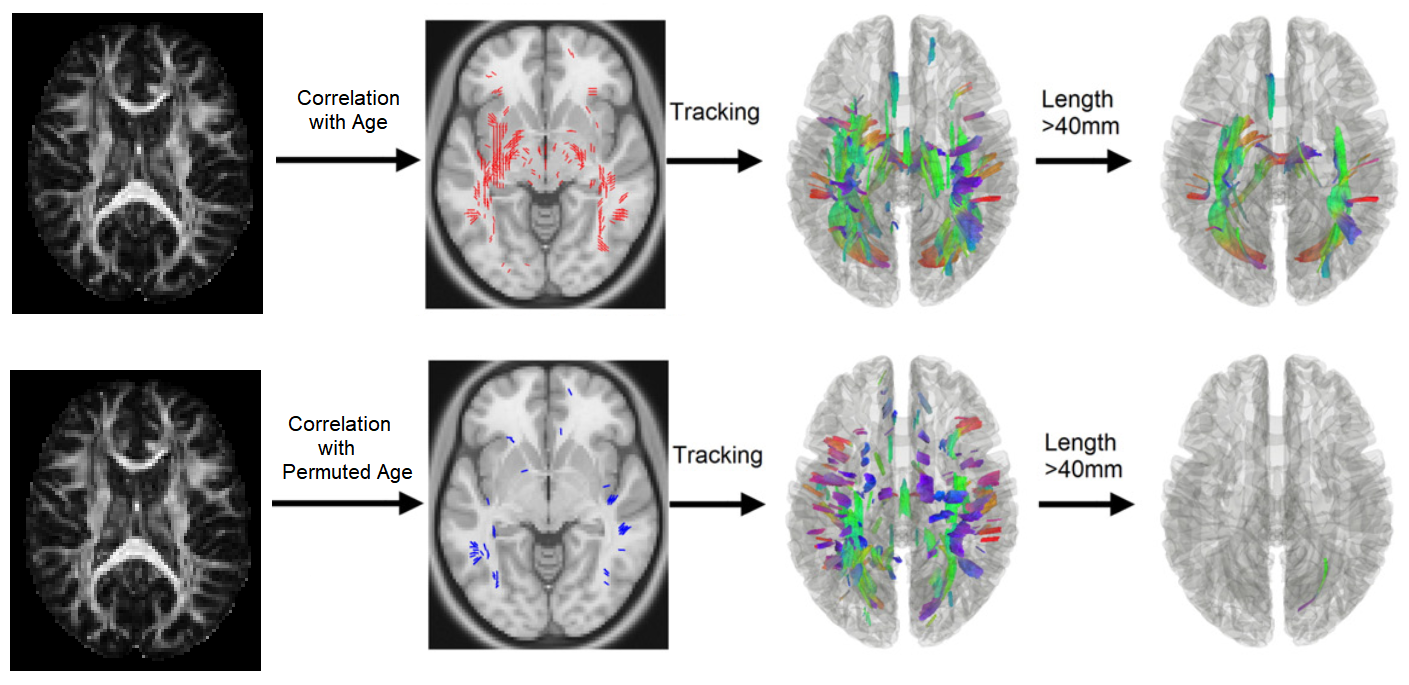# Correlational Tractography

## During practicum on Friday:

### Correlation tractography

Conventional fiber tracking Differential fiber traacking Correlational Tractography
seed point start at any white matter location start at any white matter location start at any white matter location
propagation propagate along fiber orientation propagate along fiber orientation propagate along fiber orientation
termination criteria anisotropy threshold, angular threshold anisotropy threshold, angular threshold, threshold for the anisotropy decrease anisotropy threshold, angular threshold, threshold for the t-statistics

### ConnectometryConnectometry: a statistical method using permutation test and bootstrap resampling to test the significance of correlational tractography.

### Steps

• Calculate t-statistics using Spearman rank-based correlation
• permuted versus non-permuted, respectively, both after bootstrap resampling
• Fiber tracking based on t-statistics
• Each fiber tracking from a seed is a statistical test.
• The test statisticsis the length of track.
• positive findings: length > L, negative finding: length < L
• FDR calculation at length L
• #tracts with length > L after permutation => #false positive
• #tracts with length > L without permutation => #false positive + #true positive
• FDR = (#false positive)/(#false positive + #true positive)
• Results
• Fixed L → report FDR
• FDR threshold → estimate L* → showing findings satisfying FDR threshold

### Types

• cross-sectional versus longitudinal study
• correlation with a categorical variable (e.g. control v.s. patient) or continuous variable (e.g. age)
study type correlation type examples of null hypothesis
cross-sectional correlation with a categorical variable the tractography with decreased metrics in group 1 is the same as those after group permutation.
cross-sectional correlation with a continuous variable the tractography with decreased metrics correlated with age is the same as those after age permutation.
longitudinal increased of decreased the tractography with decreased metrics is the same as those after random-permuting the sign of change.
longitudinal associated with a categorical variable the tractography with decreased metrics in group 1 is the same as those after group permutation.
longitudinal associated with a continuous variable the tractography with decreased metrics correlated with age is the same as those after age permutation.

### Hands-on: cross-sectional analysis

• correlational tractography correlated with group
• report FDR given a length threshold
• assign FDR threshold and report findings
• high t-threshold (more localized) versus low t-threshold (less localized)
• correlational tractography correlated with group under partial correlation considering age/sex.
• stratified analysis using cohort selection (e.g. male and female).
• combined ROI/ROA/terminative (wk 3 course)
• post-hoc analysis
• identifying pathways using manual virtual dissection and recognition (wk 2 course)
• tract-metric scatter plot using tract-based analysis (week 5 hw).

### Hands-on: longitudinal analysis

• compute longitudinal change using [Step C2][Tools][Longitudinal Scans]
• use connectometry to answer the following question
• are there significant decrease of FA in the patient group?
• are there significant decrease of FA in the control group?
• are decreased FA in the control group significantly correlated with age?
• are decreased FA in the patient group significantly correlated with age?
• are there significantly more changes in the patient group than control group?
• are there significantly more changes in the patient group than control group in the brainstem?